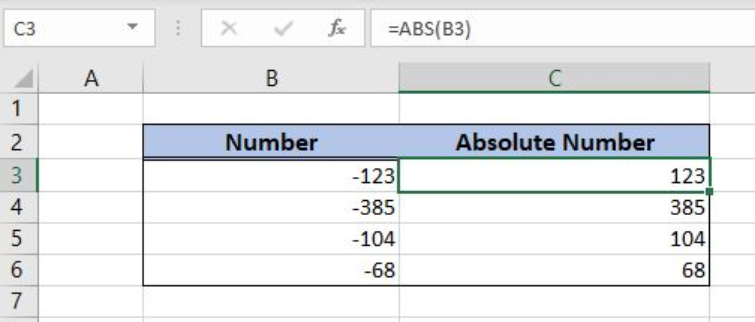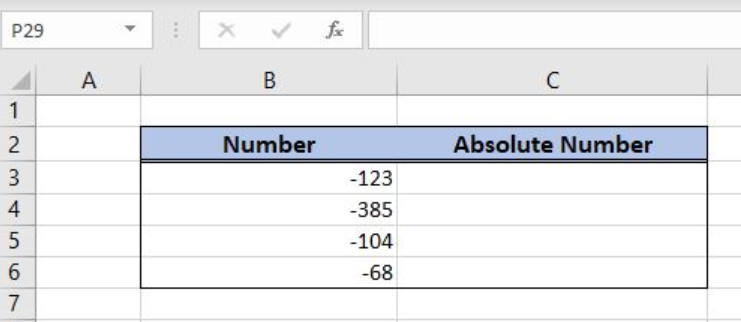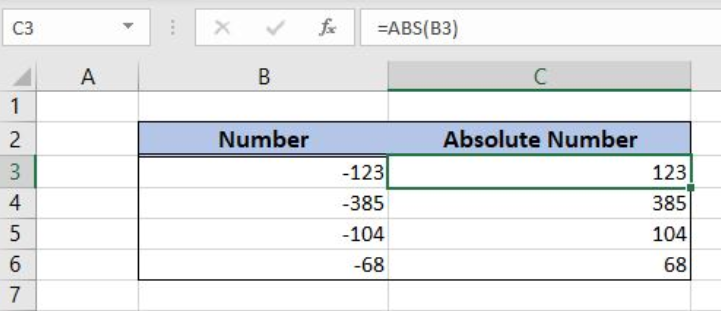Get instant live expert help with Excel or Google Sheets“My Excelchat expert helped me in less than 20 minutes, saving me what would have been 5 hours of work!”

#### Post your problem and you'll get expert help in seconds

Your message must be at least 40 characters
Our professional experts are available now. Your privacy is guaranteed.

# Excel ABS Function

Excel allows us to get an absolute value of a number, by using the ABS function. The ABS function converts a negative number to a positive This step by step tutorial will assist all levels of Excel users in getting an absolute value of a number.Figure 1. The result of the ABS function

## Syntax of the ABS Formula

The generic formula for the ABS function is:

`=ABS(number)`

The parameters of the ABS function are:

• number – a number which we want to convert from negative to positive.

## Setting up Our Data for the ABS Function

In column B (“Number”), we have the list of numbers and in column C (“Absolute number”), we want to get an absolute value for the numbers.Figure 2. Data that we will use in the ABS example

## Get an Absolute Value for a Number

In our example, we want to get absolute values for numbers from column B in column C.

The formula in the cell C3 is:

`=ABS(B3)`

The parameter number is the cell B3.

To apply the ABS function, we need to follow these steps:

• Select cell C3 and click on it
• Insert the formula: `=ABS(B3)`
• Press enter
• Drag the formula down to the other cells in the column by clicking and dragging the little “+” icon at the bottom-right of the cell.Figure 3. Using the ABS function

As you can see, the value from B3 (-123) is converted to its absolute value (123).

Most of the time, the problem you will need to solve will be more complex than a simple application of a formula or function. If you want to save hours of research and frustration, try our live Excelchat service! Our Excel Experts are available 24/7 to answer any Excel question you may have. We guarantee a connection within 30 seconds and a customized solution within 20 minutes.

### Did this post not answer your question? Get a solution from connecting with the expert.Another blog reader asked this question today on Excelchat:## Subscribe to Excelchat.coAnother blog reader asked this question today on Excelchat: